Test Description

## 40 Questions MCQ Test | JEE Advanced Test 2

 1 Crore+ students have signed up on EduRev. Have you?
JEE Advanced Test 2 - Question 1

### Which of the following expressions is true ?

JEE Advanced Test 2 - Question 2

### For the reaction, NH3(g) → 1/2N2(g) + 3/2H2(g) , the degree of dissociation in terms Kp is

JEE Advanced Test 2 - Question 3

### The pH of a solution obtained by mixing20 mL of 0.2M sodium acetate and 10 mL of 0.1 M acetic acid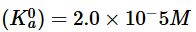is

JEE Advanced Test 2 - Question 4

At isoelectric point,

JEE Advanced Test 2 - Question 5

The aldol condensation between PhCHO and CH3COC2H5 is basic condition and in acidic conditions give respectively

JEE Advanced Test 2 - Question 6

Which of the following orders regarding acid strength of isomers of nitrobenzoic acid is correct ?

JEE Advanced Test 2 - Question 7

Which of the following statements regarding maleic acid and fumaric acid is correct ?

JEE Advanced Test 2 - Question 8

Which of the following oxides is an acidic oxide ?

JEE Advanced Test 2 - Question 9

Which of the following facts is correct ?

JEE Advanced Test 2 - Question 10

Which of the following facts regarding change in bond length is correct?

*Multiple options can be correct
JEE Advanced Test 2 - Question 11

Which of the following statements are correct ?

*Multiple options can be correct
JEE Advanced Test 2 - Question 12

Which of the following statements are correct ?

*Multiple options can be correct
JEE Advanced Test 2 - Question 13

Which of the following statements are correct ?

*Multiple options can be correct
JEE Advanced Test 2 - Question 14

The correct statement(s) concerning the structures E, F and G is (are)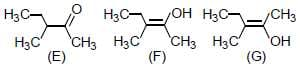*Multiple options can be correct
JEE Advanced Test 2 - Question 15

Which of the following statements are correct ?

*Multiple options can be correct
JEE Advanced Test 2 - Question 16

Which of the following statements are correct ?

*Multiple options can be correct
JEE Advanced Test 2 - Question 17

Which of the following statement(s) is(are) correct with reference to the ferrous and ferric ions ?

*Multiple options can be correct
JEE Advanced Test 2 - Question 18

For the equilibrium, , the equilibrium constant is of the order of 103. It can be concluded that

*Multiple options can be correct
JEE Advanced Test 2 - Question 19

Which of the following statements are correct ?

*Multiple options can be correct
JEE Advanced Test 2 - Question 20

An aqueous solution contains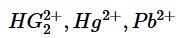and Cd2+. The addition of HCl (6N) will precipitate

JEE Advanced Test 2 - Question 21

For the reaction,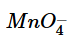+ 8H+ 5Fe2+ → Mn2+ + 4H2O + 5Fe3+ ,

it is given that, E0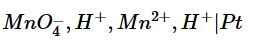= 1.51V and E0 (Fe3+ , Fe2+ | Pt) = 0.77V

The cell emf could be increased above the standard emf by

JEE Advanced Test 2 - Question 22

For the reaction,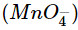+ 8H+ 5Fe2+ → Mn2+ + 4H2O + 5Fe3+ ,

it is given that, E0= 1.51V and E0 (Fe3+ , Fe2+ | Pt) = 0.77V

Reducingto 0.50 M keeping all other concentrations at unity, the emf of the cell will be changed by

JEE Advanced Test 2 - Question 23

Nitric oxide is absorbed by a cold solution of ferrous sulphate due to the formation of the dark brown nitrosyl complex ion. [Fe(NO)(H2O)5]2+.

The number of unpaired electrons in the complex ion is

JEE Advanced Test 2 - Question 24

Nitric oxide is absorbed by a cold solution of ferrous sulphate due to the formation of the dark brown nitrosyl complex ion. [Fe(NO)(H2O)5]2+.

The dark brown colour is due to

JEE Advanced Test 2 - Question 25

Ni2+ ions form the complexes [NiCl4]2- and Ni(CO)4.

Which of the following statements is correct ?

JEE Advanced Test 2 - Question 26

Ni2+ ions form the complexes [NiCl4]2- and Ni(CO)4.

Which of the following statements is correct ?

JEE Advanced Test 2 - Question 27

Match List I with List II

List I                          List II
A. E0 = 0                   p. Cell is discharged
B. E = 0                    q. Q = K
C. ΔG = 0   r. 96500 coulomb
D. 1 Faraday            s. 1 mol electrons
t. Concentration cell

JEE Advanced Test 2 - Question 28

Match List I with List II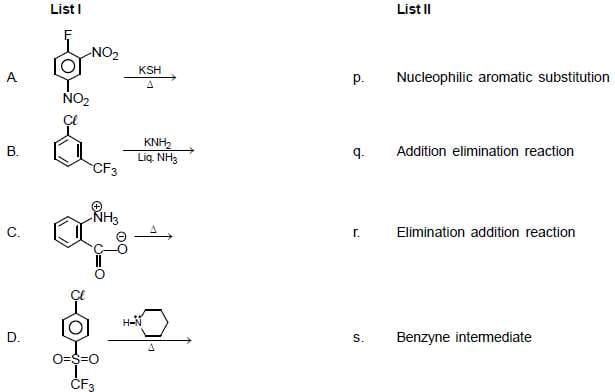JEE Advanced Test 2 - Question 29

Match List I with List II
List I                                                                                       List II
A. Real gas at high pressure                                                 p. PV = RT + Pb
B. Force of attraction among gas molecules is negligible     q. PV = nRT
C. At high temperature and low pressure                              r. Z = 1
D. Real gas at NTP                                                               s.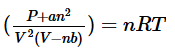JEE Advanced Test 2 - Question 30

Match List I with List II
List I                                         List II
A. NH3 + H+ → Product           1. pπ—pπ
B. H + F → Product                 2. sp3—s
C. N + N → Product                3. sp3—p
D. C + 4Cl → Product              4. p—s

*Answer can only contain numeric values
JEE Advanced Test 2 - Question 31

Find out number of benzylic hydrogen in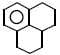*Answer can only contain numeric values
JEE Advanced Test 2 - Question 32

How many alkenes, from following are more stable than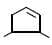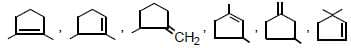*Answer can only contain numeric values
JEE Advanced Test 2 - Question 33

Find out number of stereogenic centres present in following compound ‘simvastatin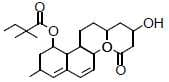*Answer can only contain numeric values
JEE Advanced Test 2 - Question 34

A cubic solid is made up of two elements P and Q. Atoms of Q are present at the corners of the cube and atoms of P at the body centre. The coordination number of P and Q will be

*Answer can only contain numeric values
JEE Advanced Test 2 - Question 35

If the rate of reaction is 2.6 x 10-3 mol L-1 s-1 at 500C and 7.02 x 10-2 mol L-1 s-1 at 800C; then what will be the temperature coefficient of the reaction ?

*Answer can only contain numeric values
JEE Advanced Test 2 - Question 36

For the equilibrium of the reaction,NH4Cl(s) → NH3 (g) + HCl(g),Kp = 81 atm2 . Total pressure equilibrium will be x times the pressure of NH3. The value of x will be

*Answer can only contain numeric values
JEE Advanced Test 2 - Question 37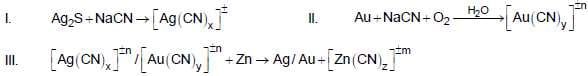Find the value of x, y and z and report your answer as th sum of x, y and z.

*Answer can only contain numeric values
JEE Advanced Test 2 - Question 38

Find the total number of non-linear species out of given species;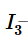,BeCl2,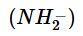,OH2,XeF2,N2O,SO2,SF2

*Answer can only contain numeric values
JEE Advanced Test 2 - Question 39

A list of species having the formula XZ4 is given below
XeF4,SF4,SiF4,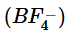,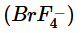,[Cu(NH3)4]2+ ,[FeCl4]2- ,[CoCl4]2- ,[PtCl4]2-
Defining shape on the basis of the location of X and Z atoms, the total number of species having a square planar
shape is

*Answer can only contain numeric values
JEE Advanced Test 2 - Question 40

Of the following reactions, how many reaction, are used for the preparation of amines.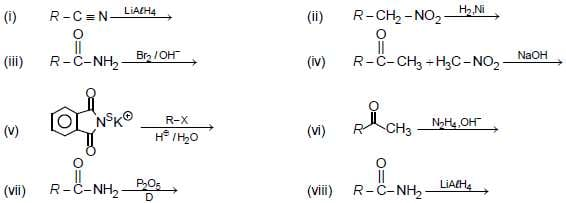(Scan QR code)